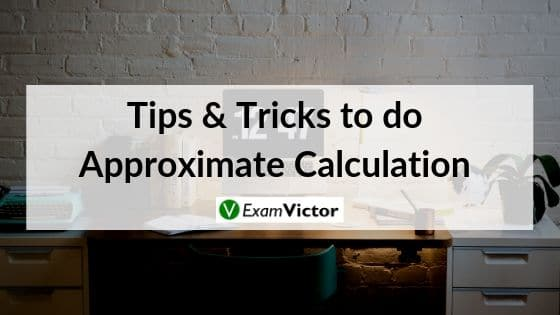# How to do Approximate CalculationLast Updated on Oct 16, 2020

The mantra to master approximate calculation is to Practice& Quick Calculation. Remember the BODMAS rule and all the Square Roots, Cube Roots & Tables should be on tips. If these things are mastered, then it is very easy to score marks in the Quant section.

One very simple form of estimation is rounding. Rounding is often the key skill you need to quickly estimate a number. This is where you make a long number simpler by ‘rounding’, or expressing in terms of the nearest unit, ten, hundred, tenth, or a certain number of decimal places.

Probably the simplest way to estimate is to round all the numbers that you are working with to the nearest 10 (or 100, if you are working in thousands at the time) and then do the necessary sum.

To approximate Actual values

If (and only if) we need to find the actual value of a given fraction, represent the numerator as sum or difference of terms related to denominator.

1449/132 =
1449 = 1320 + 132 – 3
1449/132 = 10 + 1 – a small value ≈ little less than 11 (actual value is 10.977)

36587 / 123 =
36587 = 36900 – 246 – 61.5 - …
36587 / 123 = 300 – 2 - 0.5 – a small value ≈ little less than 297.5 (actual is 297.455)

1569 / 12 =
1569 = 1200 + 360 + 8.4 + 0.6
1569 / 12 = 100 + 30 + 0.7 + 0.05 = 130.75

This method should suffice for the level of accuracy expected in our exams.

Another method is to reduce the complexity of fraction and then solve. The complexity of a fraction can be directly related to the complexity of its denominator. If we simplify the denominator, we simplify the fraction. Add to or subtract from the denominator to make it an easier value (like add 2 to 1998 to get 2000 or subtract 16 from 116 to get 100).

While adjusting the denominator always remember to BALANCE the fraction. Balancing fraction is not just adding/subtracting the same number to/from the numerator that we used to change the denominator.

Consider a fraction p/q = n; then p = qn.

If we add a number x to q, we need to add nx to p to balance the fraction. Also if q is reduced by a number x, p needs to be reduced by nx.

Here the approximation comes while fixing n. If the given options are separated well enough from each other and simplification of denominator is pretty obvious, then this method can be employed.

##### Some Tips & Tricks to do Approximate Calculation
1. Remember to have all the multiplication tables up to 20, squares up to 30, cubes up to 20, and fraction tables (1/n) on your tips.
2. Perform Average Addition, Subtraction, Multiplication & Divisions taking cases from your daily life.
3. Do not stop your practice and revision process. Continuity plays a major role in mastering something.
4. For Quick Calculations use ABACUS or Vedic Math rules.

This video lays out the basics of Making Approximate Calculations. Understanding this topic well is necessary to solve questions that are asked in various competitive exams in the Quant and DI sections - applicable to CAT, XAT, MAT, SNAP, IIFT, CLAT, AILET, DU LLB, any other entrance exam as well.